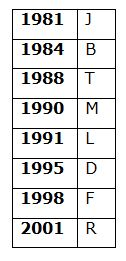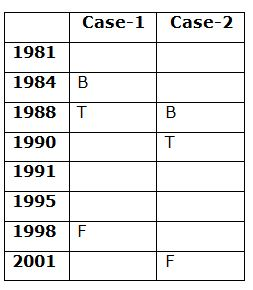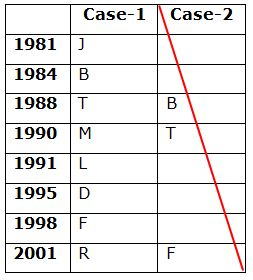# CWC/FCI Prelims 2019 Reasoning Ability Questions (Day-27)

Dear Readers, Exam Race for the Year 2019 has already started, To enrich your preparation here we are providing new series of Practice Questions on CWC/FCI Prelims 2019 Reasoning Ability Questions on daily basis. Aspirants, practice these CWC/FCI Prelims 2019 Reasoning Ability Questions (Day-20) on a regular basis to improve your score in the aptitude section. Start your effective preparation from the right beginning to get success in the upcoming CWC/FCI Exam.

[WpProQuiz 5748]

Direction (1-5): Read the following information carefully and answer the questions given below.

Eight persons B, D, F, J, L, M, R and T are born in different years viz., 1981, 1984, 1988, 1990, 1991, 1995, 1998 and 2001 but not necessary in the same order. Their ages were calculated based on the year 2019. Only three persons are born between F and T, whose age is in prime number. B was born immediately before T. D was born immediately after L. As many persons born after L is same as the persons born before M. J is not an younger than D.

1) Who among the following is the eldest person?

a) R

b) J

c) M

d) L

e) T

2) How many persons are born between M and L?

a) None

b) One

c) Two

d) Three

e) More than three

3) What is the sum of the ages of D and T?

a) 53 years

b) 59 years

c) 55 years

d) 66 years

e) None of these

4) Four of the following five are alike in a certain way and hence form a group. Which one of the following that does not belong to the group?

a) L

b) R

c) F

d) D

e) J

5) Which of the following statement is true?

a) F is elder than T

b) The age difference between D and M is 7 years

c) More than one persons born between T and J

d) Only three persons are elder than M

e) None is true

Directions (6-10): In these questions relationship between different elements is shown in the statements. These statements are followed by conclusions. You have to find out which of the conclusions follow or not from the given statements. Give answer,

(a) If only conclusion I is true

(b) If only conclusion II is true

(c) If either conclusions I or II is true

(d) If neither conclusion I nor II is true

(e) If both conclusions I and II are true

6) Statement: N≥B>G; K≤G=E; T<K≤W.

Conclusions:

I. N>T

II. W≥E

7) Statement: J<K=L≥S; B≤E<R=L≤W.

Conclusions:

I. E<J

II. S≤B

8) Statement: A=T≥C; N≤M<K; M≤A=S.

Conclusions:

I. C<K

II. S≥N

9) Statement: P>U=S≤G<K; J>H≥W≥S.

Conclusions:

I. K>H

II. H>U

10) Statement: F=D≥S>A; N≤B≥D>V=C.

Conclusions:

I. B>A

II. C<F

Direction (1-5):• Only three persons are born between F and T, whose age is in prime number. B was born immediately before T.• D was born immediately after L. As many persons born after L is same as the persons born before M.
• So, Case-2 will be dropped.
• J is not an younger than D.Directions (6-10):

I. N>T –> N≥B>G≥K>T

II. W≥E –> W≥K≤G=E

I. E<J –> E<R=L=K>J

II. S≤B –> S≤L=R>E≥B

I. C<K –> C≤T=A≥M<K

II. S≥N –> S=A≥M≥N

I. K>H –> K>G≥S≤W≤H

II. H>U –> H≥W≥S=U